Courses

# Testing Of DC Shunt Motors Electrical Engineering (EE) Notes | EduRev

## Electrical Engineering (EE) : Testing Of DC Shunt Motors Electrical Engineering (EE) Notes | EduRev

The document Testing Of DC Shunt Motors Electrical Engineering (EE) Notes | EduRev is a part of the Electrical Engineering (EE) Course Electrical Machines.
All you need of Electrical Engineering (EE) at this link: Electrical Engineering (EE)

Testing of DC Shunt Motors
In any electrical machine for that matter any equipment finding out its characteristics by actual experiment is very important, because although, it is possible to get some idea about the performance of the equipment from the design data, but experimental validation or testing is essential. This is true also for all electrical machines and particularly for DC machines. One of the important characteristics that we test for any Electrical equipment is the losses and efficiency of the equipment. The same is true for DC shunt motors.

(Refer Slide Time: 01:11)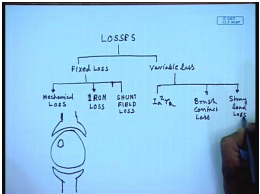In a DC machine, there is several mechanism or several components of these losses. So, losses can be broadly categorized into two parts. One is called the fixed loss or constant loss; another is called the variable loss. The fixed loss for DC shunt motor has several components; one is called the mechanical loss, assuming that the machine operates almost at constant speed then the iron loss and also the shunt field loss. Now in a DC machine although the currents and voltages are DC from outside; if we look at it, we find that in the armature if we look at any portion of the armature any volume in the armature, the flux passing through that volume is alternating, because the armature is rotating both due to field and due to armature reaction.

Therefore, there is some iron loss present in a DC machine. The iron loss is mostly due to hysteresis at Eddy current loss; hence, it has the effect of producing a opposing torque on the motion of the machine. Hence, sometimes it is represented as a part of mechanical loss. The variable loss is mostly I a square r a and the brush contact loss. In addition to this there is something called the stray load loss. There are several mechanisms that go into producing this stray load loss. For example, the skin effect of the armature conductors and then the induced iron loss in the end covers in effect of the overhang; all these items goes into make a stray load loss .

(Refer Slide Time: 04:51)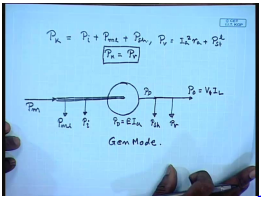So, in general the fixed loss P k of a DC machine consists of P iron plus P mechanical loss plus P shunt field loss, whereas the variable equal to I a square r a plus P stray. Maximum efficiency of this machine usually occurs when P k is equal to P V; this is the value of combination for maximum efficiency. Now let us see in the motoring and generating mode, how these losses are distributed? Let us first take the case of a generator. So, in a generator the mechanical power is input, then the first component of loss that goes out is the mechanical loss.

We said that iron loss has the effect of producing an opposing torque. So, next comes the iron loss. This is the net mechanical power input to the machine and then the developed power P D equal to E I a. So, this is P D from which you will have P shunt field loss going out. Then you will have P D going out, and finally, you will have the P O which is equal to V t I L; this is generator mode. In the motor mode the diagram will reverse to some extent.

(Refer Slide Time: 08:14)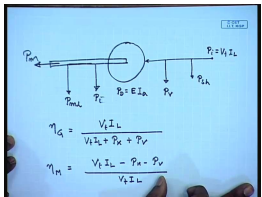So, in the motor mode the electrical power is input; the first component of loss that goes out is the shunt field loss, and then this P V. Here you get P developed is equal to E I a; from there P iron it has to overcome, and then it has to overcome mechanical losses. And finally, you will have the mechanical power output. So, the generator efficiency equal to V t I L divided by V t I L plus P k plus P v. And motor efficiency nu M equal to V t I L minus P k minus P v by V t I L. There are several testing methods available in order to find out the different components of the losses of a DC machine.

(Refer Slide Time: 10:25)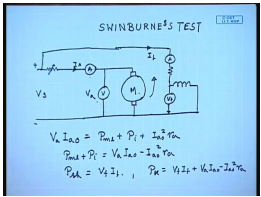One of them is called the Swinburne’s test. This is a no load test mostly to determine the components of P k. The circuit diagram is like this. You have the machine which is rotated. We have an ammeter and a voltmeter connected, and the machine is connected to the separated supply voltage through an adjustable armature resistance. And then the field is also supplied through an ammeter and an adjustable field resistance. Now this is the torque supply voltage V s, field current I f, armature current I A; this is the armature voltage V a. The machine is operated at no load with rated field applied with rated field applied; if the machine operates at no load then in the motor mode it will operate at slightly higher than the rated speed.

In order to bring down the speed to the rated value, this armature resistance is included. Now the armature reading and the voltage that it will get is the no load armature current. So, the power supplied it is V a reading of this voltmeter V a I a 0. This is basically P m l plus P i plus I a 0 square r a or P m l plus P i equal to V a I a 0 minus. Similarly, P s h equal to V f I f. So, P k equal to V f I f plus V a I a 0 minus I a 0 square r a. In order to compute P k, we need to find out what is r a. This includes also the brush contract drop. So, this Swinburne’s test usually gives the different components of the fixed losses, but since this is a no load test, the load loss components cannot be determined; particularly the stray load cannot be determined.

And since this is a cold run test, the machine temperature does not rise up to the rated value. Hence, whatever losses we get that are conservative. And since this machine is not loaded in this case, it is not possible to predict whether the commutation of the machine will be satisfactory under load condition. So, here the estimate of efficiency that we will get from this test will be somewhat on the higher side.

(Refer Slide Time: 16:09)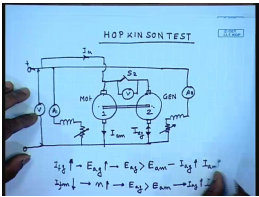Therefore, in order to overcome these problems another test method which is called the Hopkinson test is used. Hopkinson test is a load test, but also a back to back test; that is here you have two identical DC machines mechanically coupled and connected to the same supply in the DC supply. The fields of the machines are supplied from the same supply. So, this is motor, and this is generator. So, what we do? Initially, this machine one is started as a motor and brought to rated speed by adjusting the field current. Next the field excitation of the generator is also excited is adjusted so that the voltage across this switch s 2 becomes they have the same polarity and the voltage across the switch s 2 becomes 0.

Once it is 0, then this switch is closed. Now what happens is that now the total current drawn from the supply will be simply the losses of the two machine; the power developed by the machine, the power developed by the generator will be electrical power developed by the generator will be consumed by the motor, whereas the mechanical power developed by the motor will be used to run the generator. So, this is something like a back to back test which we have seen in case of a transformer which is called the Sumpner test. The cause effect relationships are like this. If the generator field current is increased, then that will cause an increase in the generated voltage.

And it will make the generated voltage of generator greater than the back E m f of the machine which will increase the generator armature current as well as the machine armature current. So, by controlling the generator field excitation we can control the loading of the two machines. Similarly, if we reduce the motor field current, this will lead to an increase in the speed of the set which will in turn increase to a generated voltage which will again make generated voltage greater than the motor back E m f which will increase the I n g and I a m. So, this way we can adjust the loading of the two machines. In order to compute the losses, we note that if this is the motor current and this is the generator current.

(Refer Slide Time: 23:05)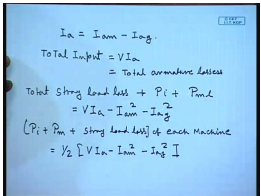Then we can write the net current drawn from the supply I a equal to I a m minus I a g. So, the total input the machine equal to V I a. So, this is equal to total armature losses since there is no output. So, the stray load loss total equal to V I a minus I a m square, total stray load loss plus P i plus P m L of both the machines; this is equal to this. So, for each machine is half of; here we are making an assumption that the machines are identical. There is no way to accurately determine the division of these losses between the two machines.

(Refer Slide Time: 26:56)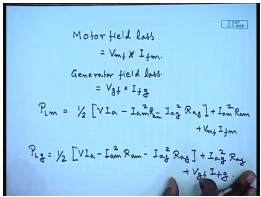So, motor field loss equal to. So, total loss in the motor equal to and similarly.

(Refer Slide Time: 30:48)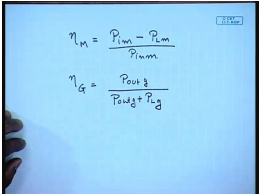The motor efficiency generator efficiency, so like Sumpner test the advantages of this Hopkinson test at that two machines are tested under loaded condition so that the stray load loss component can be taken care of. Also they can be tested at various levels of load without actually loading them; however, there are certain assumptions involved. For example, we assume that the machines are identical and the stray load loss and the iron loss, row frictional loss are equally divided into the two machines; that is one thing.

And second is that that the two machines losses are stray load loss, etcetera are assumed to be. For small machine large variation of field current is normally required to match the speeds. So, for small machine this testing does not give equal division of losses between two machines. So, this testing is more useful for larger machines rather than small machines. So, let us solve a few problems to find out how exactly we determine the losses and efficiency of DC machines by performing these tests.

(Refer Slide Time: 34:01)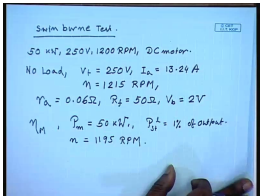So, let us first see something with the Swinburne test. We have a 50 kilowatt, 250 volt, 1200 RPM DC motor. On no load with V t equal to 250 volts, the armature current is 13.24 amperes, and the speed is 1215 RPM. The resistance measurements and brush contract drop measurement gave you r a equal to 0.06 ohm, R f equal to 50 ohm and V b equal to 2 volts; that is the drop in the brushes. So, what will be the efficiency of this motor for a shaft output of 50 kilowatt? Assume that the stray load losses are 1 percent of output. This assumption is sometime made, because it is very difficult to accurately measure this loss. The speed turns out to be at 50 kilowatt shaft load, n equal to 1195 RPM.

(Refer Slide Time: 37:03)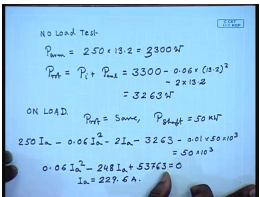So, from the no load test armature input equal to 250 volts into 13.2 ampere. This gives you 2300 watts; this is the power input at no load. So, what is the rotational losses? This is equal to iron losses plus mechanical losses. This comes to the no load power input minus the no load armature copper loss minus the brush losses. Now the speed varies from 1250 to 1995 RPM. We assume that due to this small variation speed, the rotational loss does not change.

So, on load P rotational remains same, and P shaft equal to 50 kilowatt. Let the corresponding armature current be I a. Then the input power is 250 I a minus armature resistance loss minus brush loss minus rotational losses minus stray load loss, which is assumed to be one percent of the output. This is equal to 50 kilowatt. So, rearranging terms we get I a square minus 248 I a plus 53763 equal to 0 from which we get I a equal to.

(Refer Slide Time: 41:28)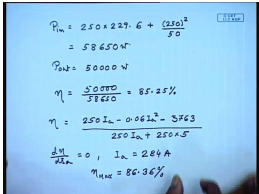So, input power P in plus the shunt field power is 250 square by 50. This comes to 58650 watts and P out equal to 50000 watt. So, efficiency is. Now if we assume that the stray load loss remains more or less constant, then actually the expression for efficiency is given by the supply voltage into armature current which is the input minus 0.06 I a square minus the 3763 which is one percent the rotational loss plus one percent of stray load loss. This is the output power at any armature current.

And the input power is 250 I a plus 250 into field current is 5 amperes. So, the maximum efficiency occurs d u d I a equal to 0. So, this gives your I a equal to 284 amperes, and the maximum efficiency comes to be about 86.36 percent. Here we have seen that for solving this problem we had to make an assumption of the stray load loss and assume that it remains more or less constant. With Hopkinson test we can experimentally find out what this stray load loss component will be.

(Refer Slide Time: 45:44)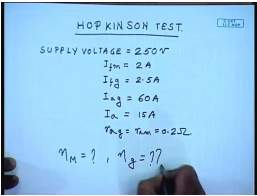So, let us solve a problem involving this Hopkinson test. In order to this Hopkinson test is performed on two machines. So, supply voltage is 250 volt. I f m is 2 ampere. I f g is equal to 2.5 amperes. I a g generator armature current equal to 60 ampere, and I a current drawn from the supply equal to 15 amperes, and r a of both the machine r a g equal to r a m equal to 0.2 ohm. Find out what is nu m, and what is nu g?

(Refer Slide Time: 47:48)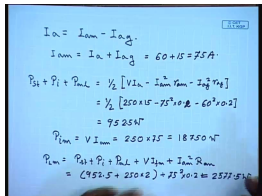The generator field current is given and we know that I a equal to I a m minus I a g; therefore, I a m equal to I a plus I a g. This comes to 60 plus 15 equal to 75 amperes. So, what is the stray load loss plus P I plus P m l of each machine. This is equal to half of V I a minus I a m square r a m minus I a g square r a g. This comes to half of 250 into I a is 15 amperes minus 75 square into 0.2 minus 60 square into 0.2 equal to 9525 watts.

The input power P in equal to V into I a m equal to 250 into 75 which is 18750 watts. So, total loss in the motor P L m equal to P s t plus P i plus P m L plus shunt field loss which is V into I f m plus I a m square R a m. This comes to 952.5 plus 250 into 2 plus 75 square into 0.2. This comes to 2577.5 watts.

(Refer Slide Time: 51:42)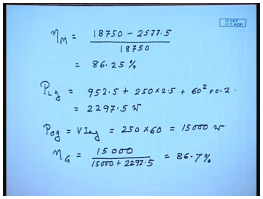So, the motor efficiency nu m equal to the input power 18750 minus losses divided by input power. This comes to 86.25 percent. Then similar term the loss in the generator comes to 952.5 plus the generator field loss with is the field current is 2.5 amperes plus generator armature current square into armature resistance. This comes to 2297.5 watts. Now generator output equal to V I a g come to 250 into 60 equal to 15000 watts. So, nu generator equal to 15000 divided by 15000 plus 2297.5. This comes to 86.7 percent.

Thank you.

Offer running on EduRev: Apply code STAYHOME200 to get INR 200 off on our premium plan EduRev Infinity!

61 docs|20 tests

,

,

,

,

,

,

,

,

,

,

,

,

,

,

,

,

,

,

,

,

,

;